• Nota de Estudos
• Rever Tópicos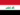Abbas A. 0 0 very nice overview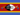Samkeliso R S. 0 0 ok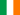Rachel N. 0 0 probability is the study of chance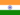Hitesh K. 0 0 this card is just a statementHitesh K. 0 0 again dice and coins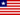Zayzay K. 0 0 Probability and relative frequency nice topic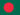Jahidul I. 1 0 Probability is the likelihood of a certain event occurring out of a total possible number of events. We can use the possible outcomes of a scenario and compare those outcomes to the desired outcome to get an idea of how likely it is that something is going to happen. You can use words like 'more likely than,' 'less likely than' and 'equally likely' to describe the probability of events. Another classical approach to probability is relative frequency, which is the ratio of the occurrence of a singular event and the total number of outcomes. This is a tool that is often used after you collect data. You can compare a single part of the data to the total amount of data collected. Edward and his friends play Pretzel and keep track of the colors that the spinner lands on.Jahidul I. 0 0 math help relative frequency ?
• Text Version### Você recebeu uma nova notificação

Clique aqui para visualizar todos eles